Question

# Simple Interest USE A 360 DAY YEAR Calculate the simple interest amount and the future value...

Simple Interest USE A 360 DAY YEAR

Calculate the simple interest amount and the future value using the simple interest formula.

365 day year

 Principal Interest Rate Time Simple Interest Amount Future Value \$ 18,000 4.5% 18 months \$ 21,000 5% 1.75 Years \$ 18,000 7.25% 9 months \$ 1,000 8% 93 days \$ 585 9% 193 days \$ 1,200 12% 187 days

1) Leslie Hart borrowed \$15,000 to pay for her child’s education. Leslie must repay the loan at the end of 9 months in one payment with 5 ½ % interest. How much interest must Leslie pay? What is the Future Value of this loan? 2) On September 12, Jody Jansen went to Sunshine Bank to borrow \$2300 at 9% interest. Jody plans to repay the loan in 137 days. How much interest will Jody owe and what is the future value of this loan? 3) Gordon Rosel went to his bank to find out how long it will take for \$1200 to increase in value to \$1650 at 8% simple interest. Please round your answer to the nearest tenth. 4) Anne Hopkins borrowed \$ 60,000. She must repay the loan at the end of 8 years in one payment with 5 ½ % interest. What will the amount of her payment be? How much of that payment is for interest?

We need at least 10 more requests to produce the answer.

0 / 10 have requested this problem solution

The more requests, the faster the answer.

All students who have requested the answer will be notified once they are available.

#### Earn Coins

Coins can be redeemed for fabulous gifts.

Similar Homework Help Questions
• ### On September 12, Jody Jansen went to Sunshine Bank to borrow \$2,300 at 9% interest. Jody...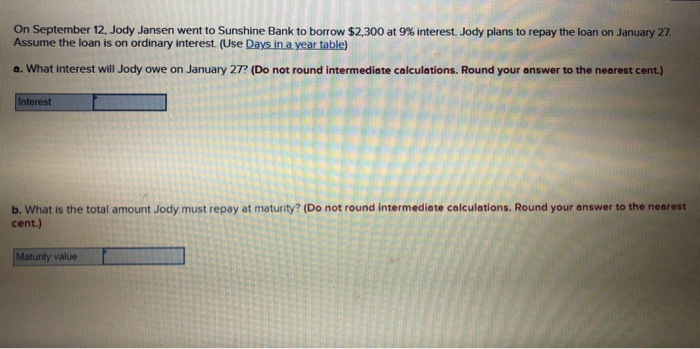On September 12, Jody Jansen went to Sunshine Bank to borrow \$2,300 at 9% interest. Jody plans to repay the loan on January 27 Assume the loan is on ordinary interest. (Use Days in a year table) a. What interest will Jody owe on January 27. (Do not round intermediate calculations. Round your answer to the nearest cent.) Interest b. What is the total amount Jody must repay at maturity? (Do not round Intermediate calculations. Round your answer to the...

• ### On September 12, Jody Jansen went to Sunshine Bank to borrow \$200 at 6% interest Jody...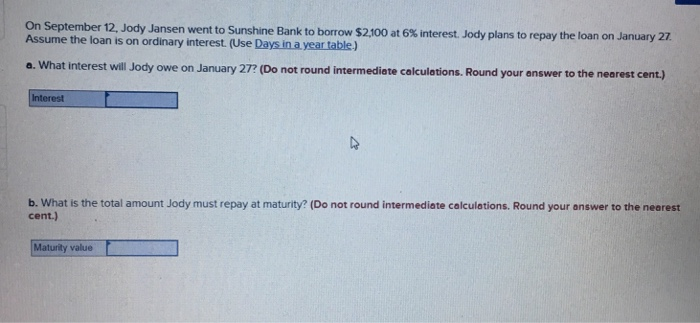On September 12, Jody Jansen went to Sunshine Bank to borrow \$200 at 6% interest Jody plans to repay the loan on January 27 Assume the loan is on ordinary interest. (Use Days in a year table) a. What interest will Jody owe on January 27? (Do not round intermediete calculetions. Round your answer to the nearest cent) b, what is the total amount Jody must repay at maturity? (D。not round intermediate calculations. Round your answer to the nearest cent.)...

• ### On September 20, Jody Jansen went to Sunshine Bank to borrow \$3,900 at 5% interest

On September 20, Jody Jansen went to Sunshine Bank to borrow \$3,900 at 5% interest. Jody plans to repay the loan on January 27. Assume the loan is on ordinary interest. (Use table value) (a)What interest will Jody owe on January 27? (Use 360 days a year. Do not round intermediate calculations. Round your answers to 2 decimal places. Omit the "\$" sign in your response.)b)What is the total amount Jody must repay at maturity? (Do not round intermediate calculations....

• ### Leslie Hart borrowed \$15,900 to pay for her child’s education at Riverside Community College

Leslie Hart borrowed \$15,900 to pay for her child’s education at Riverside Community College. Leslie must repay the loan at the end of 6 months in one payment with interest. (a)How much interest must Leslie pay? (Do not round intermediate calculation. Round your answer to 2 decimal places. Omit the "\$" sign in your response.)(b)What is the maturity value? (Do not round intermediate calculation. Round your answer to 2 decimal places. Omit the "\$" sign in your response.)

• ### gordon rosel went to his bank to find out how long it will take for \$2,300 to amount to \$2, 860 simple interest

gordon rosel went to his bank to find out how long it will take for \$2,300 to amount to \$2, 860 simple interest.

• ### Calculate the value of x. Interest Amount Principal / Present Value Interest Rate (per year) Time...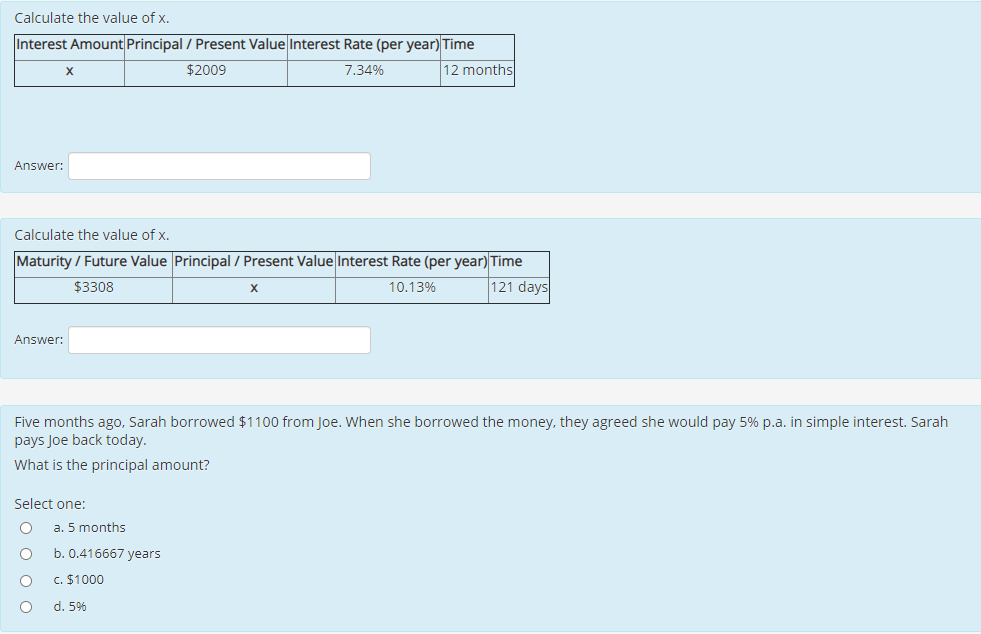Calculate the value of x. Interest Amount Principal / Present Value Interest Rate (per year) Time \$2009 7.34% 12 months х Answer: Calculate the value of x. Maturity / Future Value Principal / Present Value Interest Rate (per year) Time \$3308 10.13% 121 days Answer: Five months ago, Sarah borrowed \$1100 from Joe. When she borrowed the money, they agreed she would pay 5% p.a. in simple interest. Sarah pays Joe back today. What is the principal amount? Select one:...

• ### Gordon Rosel went to his bank to find out how long it will take for \$1,900 to amount to \$2,420 at 7.8% simple interest

Gordon Rosel went to his bank to find out how long it will take for \$1,900 to amount to \$2,420 at 7.8% simple interest. Please solve Gordon’s problem. (Round your answer to 1 decimal place.)

• ### Show all yourwork! 1) A bank borrows \$1,000,000 for 1 month at a simple interest rate...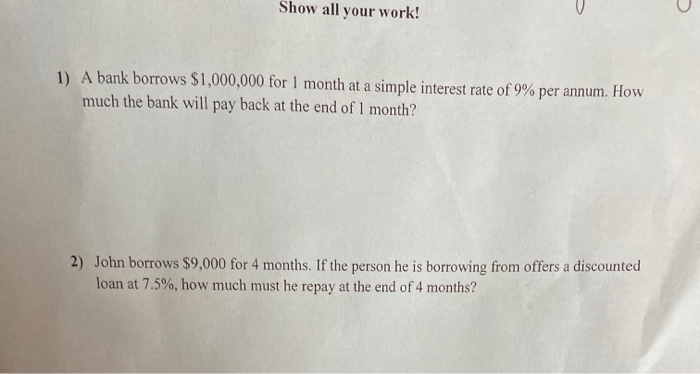Show all yourwork! 1) A bank borrows \$1,000,000 for 1 month at a simple interest rate of 9% per annum. How much the bank will pay back at the end of 1 month? 2) John borrows \$9,000 for 4 months. If the person he is borrowing from offers a discounted loan at 7.5%, how much must he repay at the end of 4 months?

• ### -.161 The amount of a certain radioactive material (in grams) in a storage facility at time...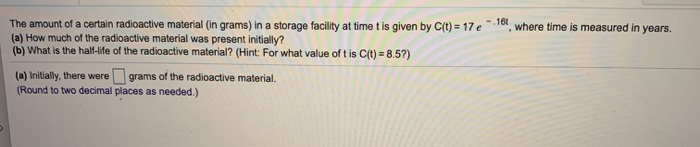-.161 The amount of a certain radioactive material (in grams) in a storage facility at time t is given by c(t) = 17 e where time is measured in years. (a) How much of the radioactive material was present initially? (b) What is the hall-life of the radioactive material? (Hint: For what value oft is c(t) = 8.5?) (a) Initially, there were grams of the radioactive material. (Round to two decimal places as needed.) Find the interest on the following...

• ### Assume the loan on exact interest. How much will Jim repay on March 11? On May 6, Jim Ryan borrowed \$14,000 from Lane B...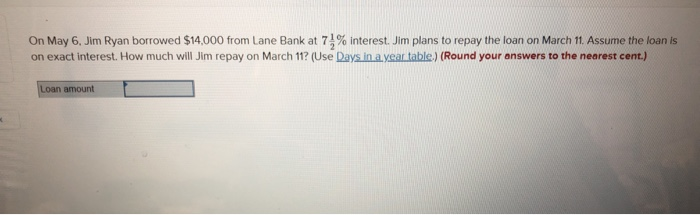Assume the loan on exact interest. How much will Jim repay on March 11? On May 6, Jim Ryan borrowed \$14,000 from Lane Bank at 7 % interest. Jim plans to repay the loan on March 11. Assume the loan is on exact interest. How much will Jim repay on March 11? (Use Days in a year table.) (Round your answers to the nearest cent.) Loan amount